Russell A. Bonham

## Some Integrals Involving Symmetric-Top EigenfunctionsNBCDFPDF

Using closure over a complete set of rotational states for methane to evaluate the intensity for quasi-elastic electron scattering in the first Born approximation, a number of integrals were encountered that appear not to have been evaluated previously. Mathematica was employed to evaluate these and similar integrals, and it was discovered that in all the cases studied the results could be represented by simple formulas.

### Details of the Integral Evaluations

The integrals discussed here are related to averages over the spherical matrix elements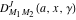[1, 2] defined by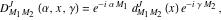(1)

which are symmetric-top eigenfunctions with eigenvalues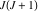and a degeneracy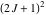. The function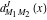is defined [1, 2] as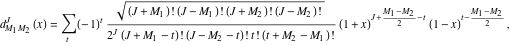(2)

where the sum is over all integer values offrom 0 to the first negative factorial that occurs in the denominator. In the impulse approximation for quasi-elastic electron scattering , averages over the rotational motion of a spherical top molecule such as methane are integrals of the following form: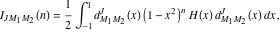(3)

where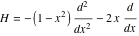(4)

is the Hamiltonian operator whose eigenfunctions are the Legendre polynomials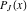with eigenvalues. Equation (4) is also a part of the Hamiltonian for which the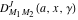are solutions, but theare not eigenfunctions of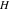(). The occurrence ofarises in the treatment of the scattering problem in the impulse approximation . By use of Mathematica it was possible to prove that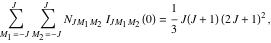(5)

where the normalization factor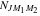is given by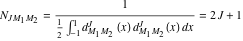(6)

for all values of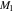and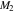. For integer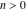the results are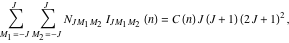(7)

where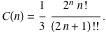(8)

The use of the word “prove” is based on the results from a Mathematica program. All of these expressions occur as ratios of positive integers and were found to be in exact agreement with the results on the right-hand sides in equation (7) for values offrom 0 through 18.

Note that the same result can be obtained for the case where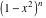is replaced by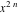if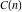is replaced by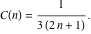(9)

Except for the factor of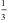for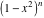and forin the definitions of the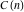, the remaining parts are equivalent to the integrals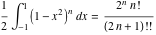(10)

and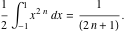(11)

Further investigation using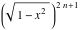found the same result with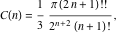(12)

where it is noted that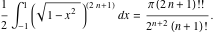(13)

Define the double sum over the integral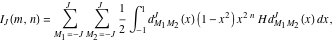(14)

and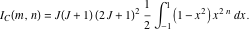(15)

These results suggest the conjecture that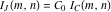, where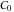is a constant. For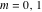and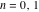, the constant factorwas found to befor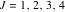, as shown in the next section.

### Implementation

The function d is.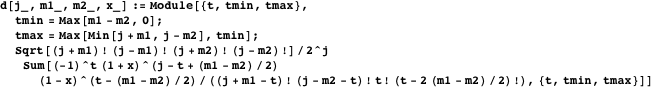The functions d1 and d2 are the first and second derivatives ofwith respect to.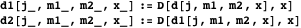The function h is the result of the Hamiltonianoperating on d.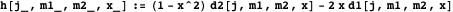Define the integral a.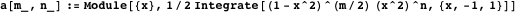The function int1 is the integral in equation (14).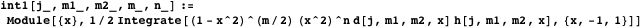The function int2 is the normalization integral of the square of.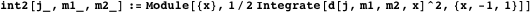The function sis the sum of the ratios of the integrals int1 and int2 overand.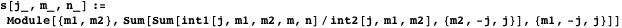For various choices of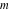,, and, the table compares the exact evaluation of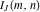(equation (14)) with the proposed result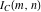(equation (15)) and shows that the ratio of the two is a constant, which in this case is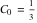. The evaluation of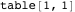takes some time.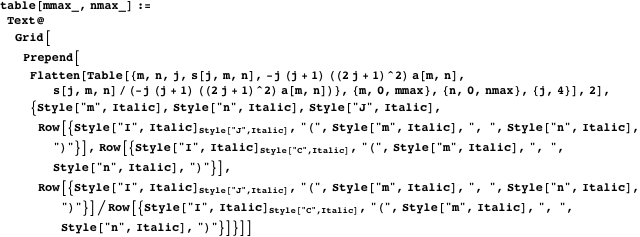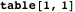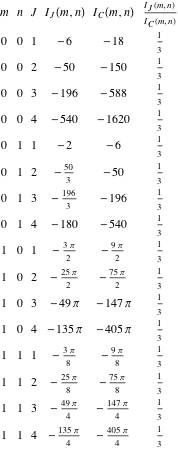### References

  D. M. Brink and G. R. Satchler, Angular Momentum, Oxford: Clarendon Press, 1979 pp. 21-25.  R. A. Bonham, G. Cooper, and A. P. Hitchcock, “Electron Compton-like Quasielastic Scattering from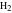,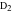, and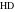,” Journal of Chemical Physics, 130 144303, 2009. dx.doi.org/10.1063/1.3108490.  G. I. Watson, “Neutron Compton Scattering,” Journal of Physics: Condensed Matter, 8(33) 5955, 1996. iopscience.iop.org/0953-8984/8/33/005. R. A. Bonham, “Some Integrals Involving Symmetric-Top Eigenfunctions,” The Mathematica Journal, 2012. dx.doi.org/doi:10.3888/tmj.14-12.